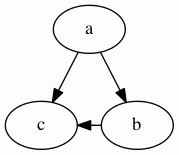# constraint

type: bool, default: `true`

If false, the edge is not used in ranking the nodes. For example, in the graph:

``````digraph G {
a -> c;
a -> b;
b -> c [constraint=false];
}``````

the edge `b -> c` does not add a constraint during rank assignment, so the only constraints are that `a` be above `b` and `c`, yielding the graph:Valid on:
• Edges
Note: dot only